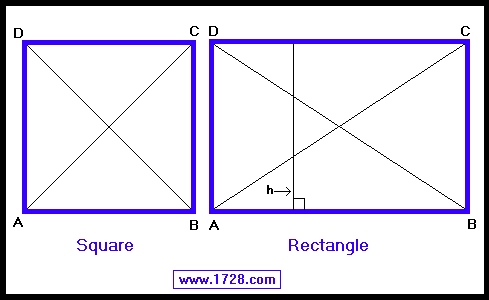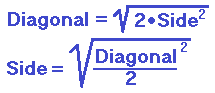Square and Rectangle Calculator
Scroll down for Instructions and Definitions

Scroll down for equations

 Click on the data you know:     SQUARE - 1 SIDE     SQUARE - 1 DIAGONAL     RECTANGLE - 2 SIDESFirst, click on one of the 4 choices, enter the data in the appropriate input boxes, then click "CALCULATE".

This calculator displays a huge amount of data for a small amount of input. For example, just by inputting the diagonal of a square (choice #2) your output will show the area of the square, the perimeter, the length of 1 side, and so on.The Square and the Rectangle

All angles equal 90°.
Lines AC and BD are called diagonals.
The diagonals are equal.
The diagonals bisect each other.
Each diagonal forms 2 pairs of congruent triangles.
Δ ADC = Δ BCD   and   Δ ACB = Δ BDA

SQUARE• DIAGONALS MEET AT RIGHT ANGLES
• ALL FOUR SIDES EQUAL

RECTANGLE
• BOTH pairs of opposite sides are equal.
AB = DC   and   AD = BC
• Line 'h' is perpendicular to lines AB & DC and is called the height or altitude.

Significant Figures >>>
The default setting is for 5 significant figures but you can change that by inputting another number in the box above.

Answers are displayed in scientific notation and for easier readability, numbers between .001 and 1,000 will be displayed in standard format (with the same number of significant figures.)
The answers should display properly but there are a few browsers that will show no output whatsoever. If so, enter a zero in the box above. This eliminates all formatting but it is better than seeing no output at all.

_____________________